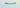# Removing first and last character from a string in JavaScriptIn this tutorial, we are going to learn about how to remove the first and last character from a string in JavaScript.

Consider we have a string like this and we need to remove the slashes from both sides.

``let str = '/hello/';``

## Using slice method

To remove the first and last character from a string, we need to specify the two arguments in slice method which are `startIndex` and `endIndex`.

``````let str = '/hello/';

//slice starts from index 1 and ends before last index
console.log(str.slice(1,-1));

//output --> 'hello'``````

Note: Negative index `-1` is equivalent to `str.length-1` in slice method.

## Using substring method

The `substring()` method also works similar like `slice` method but in `substring` negative indexes are treated as `0` so that we need to use `str.length-1` to get the `endIndex`.

``````let str = '/hello/';

console.log(str.substring(1,str.length-1));

//output --> 'hello'``````

## Using both slice and substring methods

Now we are chaining the both `slice()` and `substring()` methods to remove the first and last character from a string.

``````let str = '/hello/';

console.log(str.substring(1).slice(0,-1));

//output --> 'hello'``````

In the above code, we first remove the `first` character from a string using `substring()` method then we chain it to `slice()` method to remove the last character.

## Using replace method

The `replace` method takes two arguments, the first argument is a `regular` expression and the second argument is `replacement`.

Example:

``````let str = '/hello/';

console.log(str.replace(/^(.)|(.)\$/g,''));

//output --> 'hello'``````